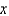# Quiz 6: Medications From Powders and Crystals: Oral and Intramuscular

Psychology

2 mL Have Want to Have 250,000 units : 1 mL :: 500,000 units :mL 25= 1 50 = 50= 2 mL Proof: 1 50 = 50 25 2 = 50

8 mL Have Want to Have 5 mL : 125 mg ::mL : 200 mg 125= 5 200 = 1000= 8 mL Proof: 5 200 = 1000 125 8 = 1000

133 mL per hour Know Want to know 10 mL : 4 g :: x mL : 2 g 4x = 10 2 = 20 x = 5 mL. Withdraw 5 mL from the vial and inject into 200 mL D5W. Proof: 10 2 = 20 4 5 = 20 Know Want to know 20 9 200 mL : 90 min :: x mL : 60 min 9x = 20 60 = 1200 x = 133.3 = 133 mL per hour to set the infusion device Proof: 20 60 = 1200 9 133.3 = 1199.9